### Home > PC > Chapter 7 > Lesson 7.1.1 > Problem7-12

7-12.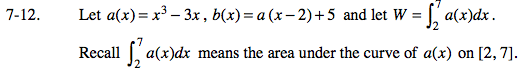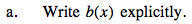Substitute (x − 2) for every x in a(x). Then add 5.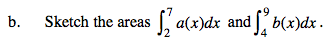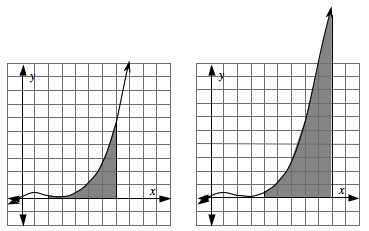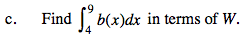The Integral is moved 2 to the right. But b(x) is also moved 2 to the right of a(x). So that is a wash. But b(x) is moved up 5. So it has more area by its width × 5. In this case, the width is 5. So if W is the area under the 'a' curve, then W + 25 is the area under the 'b' curve.